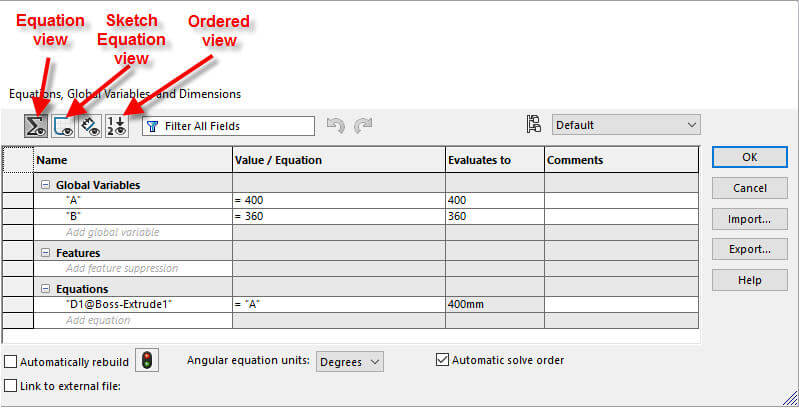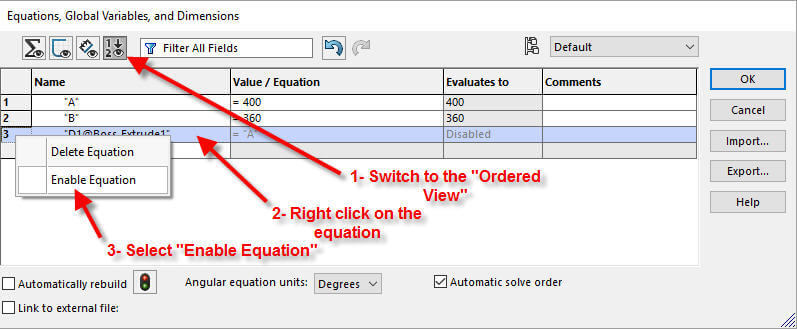# SOLIDWORKS 2017 Disabling and Enabling Equations in All ConfigurationsArticle by Saeed Mojarad (CSWE) updated October 27, 2016

###### Article

During the past few years SOLIDWORKS has improved “Equation” functionality. Up to SOLIDWORKS 2013, you could only configure equations and global variables by suppressing or unsuppressing them in selected configurations.

With SOLIDWORKS 2014 the way formulas and suppression are handled in SOLIDWORKS changed. Now you could create variations of equations and global variables, and apply them to configurations using the “Equations” and “Modify” dialog boxes. You could also configure equations and global variables in design tables, and in various property managers for parts and assembly features.

With the introduction of SOLIDWORKS 2015 you could use equations to vary pattern dimensions.

## SOLIDWORKS Equation Views

SOLIDWORKS 2017 has improved the “Equation” one more time. Now you can disable and enable an equation in the Equation View, Sketch Equation View, or Ordered View; in a variety of dialog boxes, including the Equations, Global Variables, and Dimensions dialogs. This is great functionality especially when you want to troubleshoot or apply and test different equations.SOLIDWORKS Equation Views

### How to disable an equation

To disable an equation, in any view, right-click on it and click “Disable Equation”. The equation disappears from the view.Disabling an equation in “Equation View”

### How to enable an equation

“Ordered View” is the only view that retains all equations. So, if you want to enable an equation you have to switch to “Ordered View”, find the equation, right click on it and select “Enable Equation”.Enabling an equation in “Ordered View”

#### Limitations

Now there are some limitations when you want to disable and enable equations.

• The equations are disabled in all configurations. So you cannot disable an equation in only one or some of the configurations.
• You can enable the equations if no other active equation controls the same parameter.

### SOLIDWORKS 2017 Resources

Access our resources page to get everything you need to learn what’s new in SOLIDWORKS 2017, including tech tips, demonstrations, and upcoming product webinars.#### Get Certified SOLIDWORKS Services from Javelin